# 【Python学习笔记】21：numpy傅里叶变换

### 一个简单的傅里叶变换

>>> import numpy as np
>>> import matplotlib.pyplot as plt
>>> x=np.linspace(0,2*np.pi,50)
>>> x
array([ 0.        ,  0.12822827,  0.25645654,  0.38468481,  0.51291309,
0.64114136,  0.76936963,  0.8975979 ,  1.02582617,  1.15405444,
1.28228272,  1.41051099,  1.53873926,  1.66696753,  1.7951958 ,
1.92342407,  2.05165235,  2.17988062,  2.30810889,  2.43633716,
2.56456543,  2.6927937 ,  2.82102197,  2.94925025,  3.07747852,
3.20570679,  3.33393506,  3.46216333,  3.5903916 ,  3.71861988,
3.84684815,  3.97507642,  4.10330469,  4.23153296,  4.35976123,
4.48798951,  4.61621778,  4.74444605,  4.87267432,  5.00090259,
5.12913086,  5.25735913,  5.38558741,  5.51381568,  5.64204395,
5.77027222,  5.89850049,  6.02672876,  6.15495704,  6.28318531])
>>> wave=np.cos(x)
>>> transformed=np.fft.fft(wave) #傅里叶变换
>>> plt.plot(transformed) #绘制变换后的信号
Warning (from warnings module):
File "D:\Program Files\Python\lib\site-packages\numpy\core\numeric.py", line 531
return array(a, dtype, copy=False, order=order)
ComplexWarning: Casting complex values to real discards the imaginary part
[<matplotlib.lines.Line2D object at 0x000000000622FA20>]
>>> plt.show()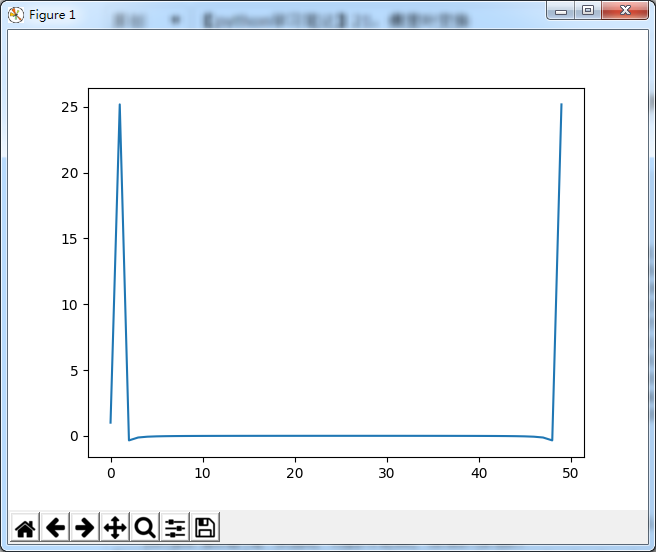### 傅里叶反变换

>>> plt.plot(np.fft.ifft(transformed)) #反变换
[<matplotlib.lines.Line2D object at 0x00000000074F0630>]
>>> plt.show()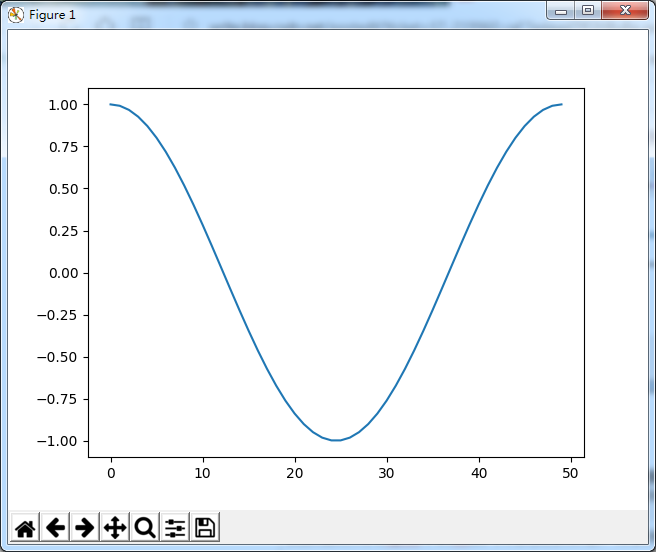### 移频(针对作好傅里叶变换的数据)

>>> shifted=np.fft.fftshift(transformed) #移频
>>> plt.plot(shifted)
[<matplotlib.lines.Line2D object at 0x00000000078AF7B8>]
>>> plt.show()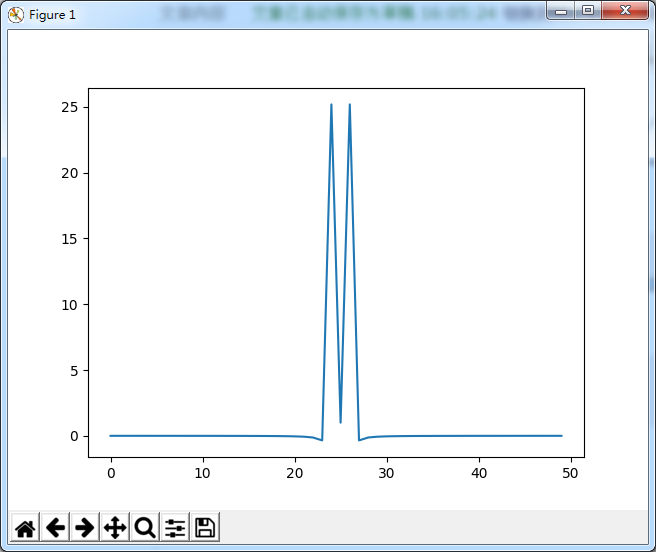### 对移频后的信号进行傅里叶反变换

>>> plt.plot(np.fft.ifft(shifted))
[<matplotlib.lines.Line2D object at 0x0000000007918DD8>]
>>> plt.show()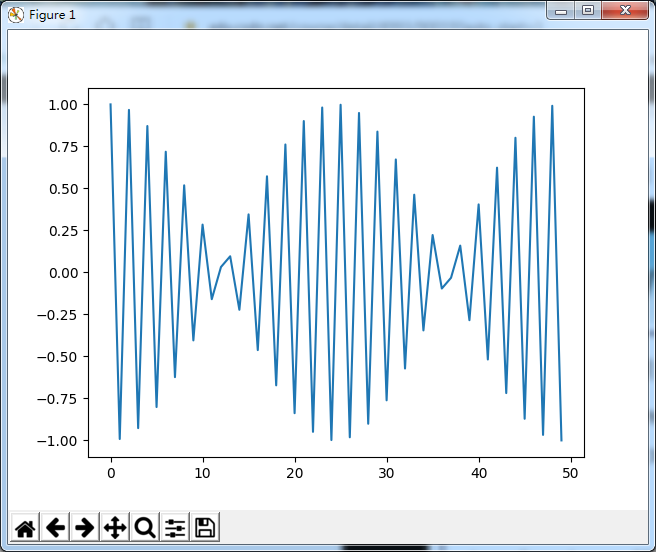### 二维傅里叶变换

>>> import numpy as np
>>> import matplotlib.pyplot as plt
>>> x=np.random.rand(10,10) #二维随机信号
>>> wave=np.cos(x)
>>> plt.plot(wave)
>>> plt.show()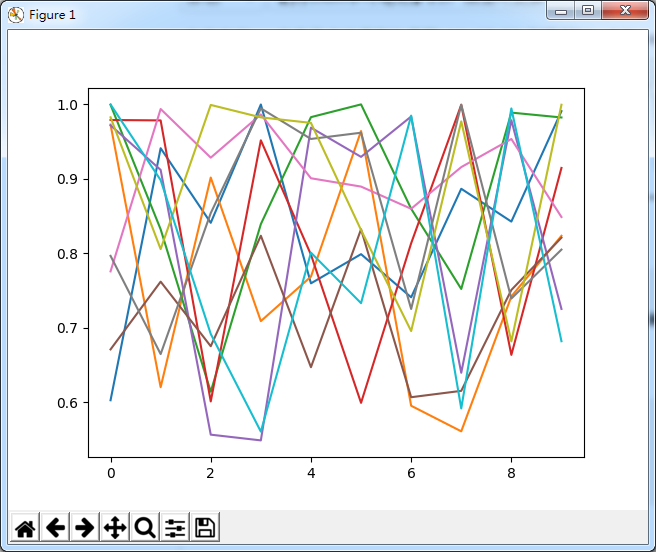>>> transformed=np.fft.fft2(wave)
>>> plt.plot(transformed)
>>> plt.show()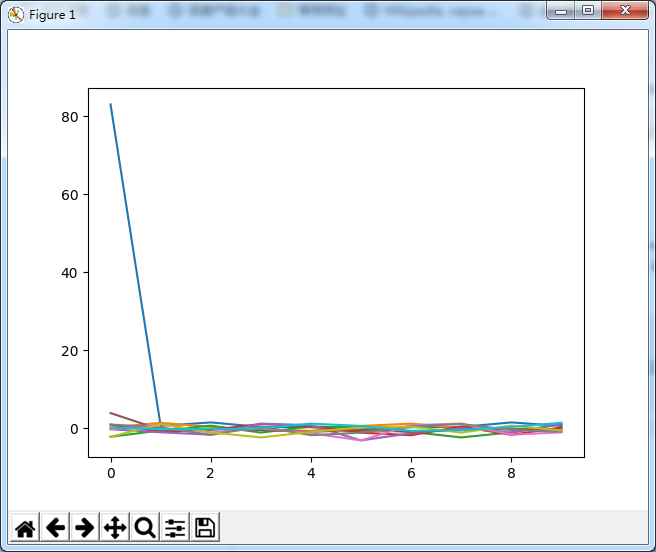>>> plt.plot(np.fft.ifft2(transformed)) #二维反变换
>>> plt.show()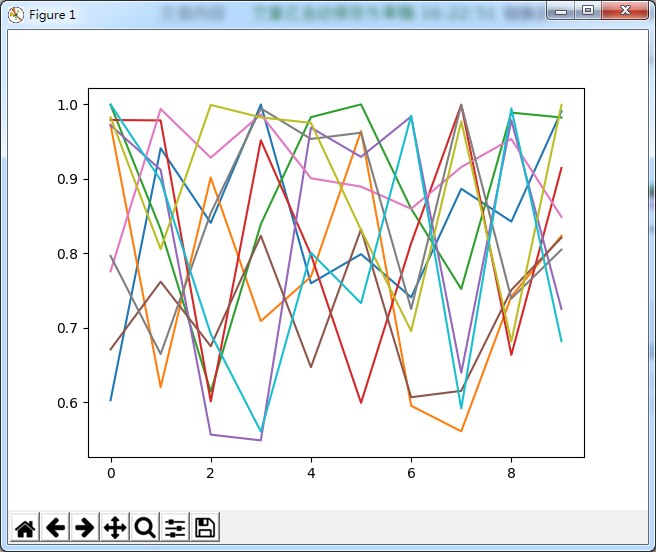### 滤出图像中的低频信号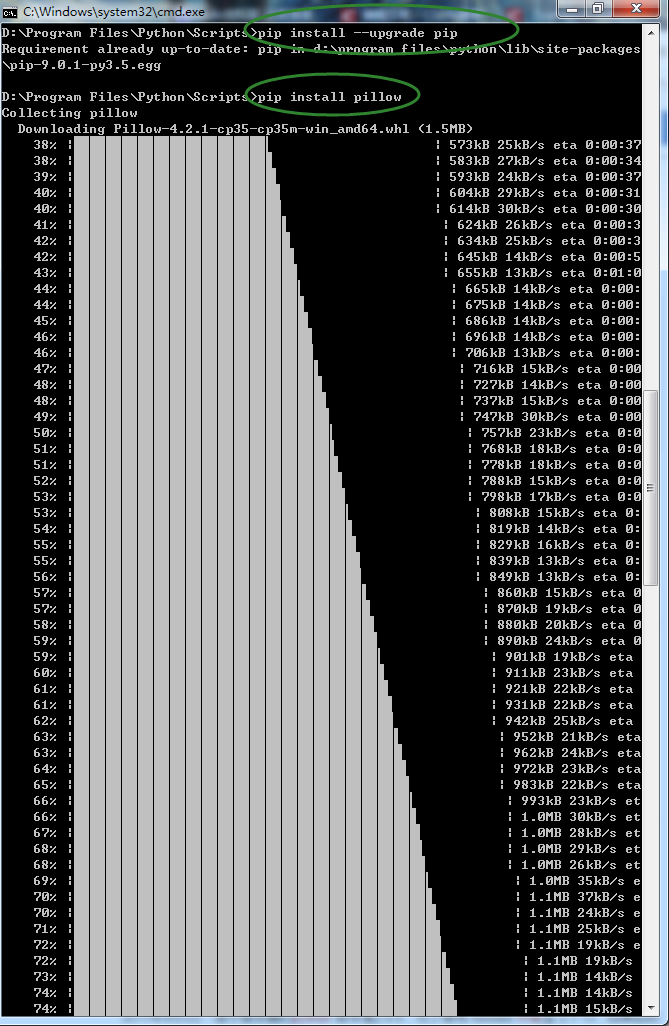import numpy as np
from PIL import Image
from numpy.fft import fft,ifft
def filterImage(srcImage):
#打开图像文件并获取数据
srcIm=Image.open(srcImage)
srcArray=np.fromstring(srcIm.tobytes(),dtype=np.int8)
#傅里叶变换并滤除低频信号
result=fft(srcArray)
result=np.where(np.absolute(result)<9e4,0,result)
#傅里叶反变换,保留实部
result=ifft(result)
result=np.int8(np.real(result))
#转换为图像
im=Image.frombytes(srcIm.mode,srcIm.size,result)
im.show()

filterImage('test.jpg')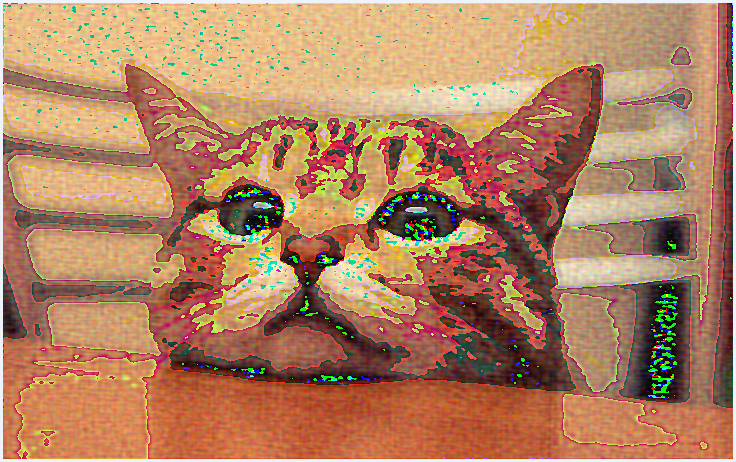04-036万+02-276303
08-20178
03-146万+
01-201万+
01-251万+
09-27775
07-11104
03-314万+
03-01157
06-151万+
06-17631
11-243623
04-176231
08-073611
08-241万+